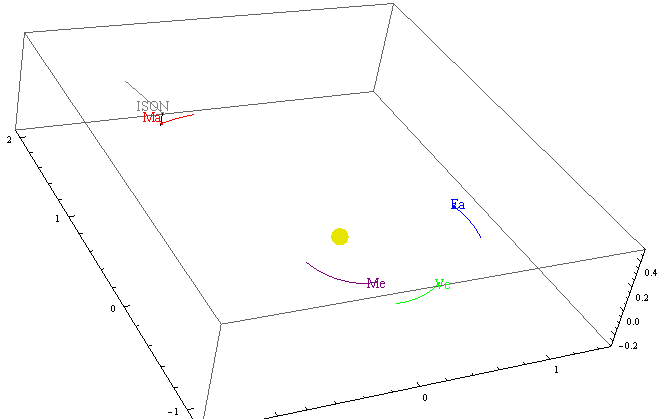# Astronomy

You are currently browsing the archive for the Astronomy category.

If you have two planets in circular orbits with semi-major axes $a_1$ and $a_2$, and periods $T_1$ and $T_2$, then the amount of time spent in retrograde is $$T_\mathrm{retro} = T_1\left|\frac{\cos ^{-1}\left(\frac{\sqrt{r}+1}{r+\frac{1}{\sqrt{r}}}\right)}{\pi\left(1-\frac{1}{r^{3/2}}\right)}\right|$$

where $r= \frac{a_2}{a_1}$. You can apply this formula to the planets in our solar system to get approximations for their time in retrograde as seen from Earth. (These approximations are often within 10% of the correct value, but sometimes the error is larger.)

$$\begin{array}{ccc} & \text{a in AU} & T_{\text{retro}} \\ \text{Mercury} & 0.387 & 23 \text{ days} \\ \text{Venus} & 0.723 & 42 \text{ days} \\ \text{Mars} & 1.524 & 73 \text{ days} \\ \text{Jupiter} & 5.204 & 121 \text{ days} \\ \text{Saturn} & 9.54 & 138 \text{ days} \\ \text{Uranus} & 19.19 & 152 \text{ days} \\ \text{Neptune} & 30.05 & 158 \text{ days} \\ \end{array}$$

It’s interesting to note that:

• $\lim_{r\rightarrow\infty} T_\mathrm{retro}(r) = T_1/2$,
• $\lim_{r\rightarrow 1} T_\mathrm{retro}(r) = T_1\cdot \frac{\sqrt{2}}{3 \pi }$, and
• $\lim_{r\rightarrow 0} \frac{T_\mathrm{retro}(r)}{r^{3/2}T_1}=1/2$. If people are interested, I can post a derivation.

If people are interested, I can post a derivation.

## John Dobson, 1915–2014

In September 2008, I met John in Harrisburg.  We slept overnight at his friend Zoungy’s place and then enjoyed the beautiful drive to Cherry Springs for the Black Forest Star Party.  John was perhaps the most entertaining, charming, and inspiring person I have ever met.  He had a million stories about astronomy, philosophy, science, and life in general.  At age 93, he was recovering from a stroke, meeting new people, travelling alone with strangers like me, and giving entertaining informative speeches to crowds numbering in the hundreds.  He would charm everyone he met.

John — rest in peace.

http://www.skyandtelescope.com/news/home/John-Dobson-1915ndash2014-240456881.html

http://www.astronomy.com/news/2014/01/astronomy-popularizer-john-dobson-dies

## Comet ISON passing Mars todayComet ISON will pass Mars at around 1600 GMT (noon in the eastern USA) today at a distance of about 7 million miles.  Unfortunately, it is right beside the moon this morning in Leo, so it would be quite difficult to see even with a telescope.  (The moon will be out-of-the-way after Oct 5).

I made a video and a Mathematica demonstration showing the path of ISON through the solar system, but there is a much nicer interactive viewer at solarsystemscope.com.

## Comet ISON, Perihelion, Mars, and the rule of 13.3

Comet Ison passing Mars (Wolfram Alpha)

I just got back from the Black Forest Star Party where Dr. Carey Lisse, head of NASA’s ISON Observing Campaign, gave a speech on comet ISON.

Comet ISON (see , ) is passing by Mars soon (Oct 1) and it will be “grazing the sun” before the end of the year, so I wondered if there was some relationship between the orbital period of a planet and the time it takes a passing comet to go from the planet to the Sun. Turns out there is a relationship.  Here’s the approximate rule:

Time to sun $\approx$ Orbital Period / $3 \pi \sqrt{2} \approx$  Orbital Period / 13.3 !

In the case of ISON and Mars,

Time to sun $\approx$ 687 days / 13.3 $\approx$ 52 days.

But Oct 1 + 52 days is Nov 22, and perihelion is on Nov 28.  Why so far off?

Well, turns out that Mars is farther from the sun than usual.  If we correct for that, then the formula estimates perihelion to within 1 day—much better.

For those that like derivations, here is the derivation for the 13.3 rule.

The orbital period of a planet is $T_p = 2 \pi \sqrt{ r^3 \over {G m_S} }$ where $m_S$ is the mass of the Sun, $r$ is the radius of the planet’s orbit (or, more precisely, the semi-major axis of its orbit), and G = 6.67e-11 is the gravitational constant.

The speed of a comet from the Oort cloud is derived from its energy.

Kinetic Energy = -Potential Energy

$\frac12 m v^2 = G m m_S / r$

$v = \sqrt{ {2 G m_S}\over{r}}$

where $r$ is the distance from the comet to the sun.

So the time for a Sun grazer to go from distance $r_0$ to the sun is about

$$T_S = \int_0^{r_0} {1\over v} dr$$

$$= \int_0^{r_0} \sqrt{ r\over{2 G m_S}} dr$$

$$= \frac13 \sqrt{ 2 r^3 \over{G m_S} }.$$

Finally,

$$T_p/T_S = {{2 \pi \sqrt{ r^3 \over {G m_S} }}\over{ \frac13 \sqrt{ 2 r^3 \over{G m_S} }}} = 3 \pi \sqrt{2} \approx 13.3.$$

## A New Dalton Minimum ? (Off topic)Seemingly weak Solar Cycle #24

A long time ago I was a meteorologist at the Joint Typhoon Warning Center in Guam.  One of my forecasters had a friend that used solar images to forecast wheat futures.  I wonder what those people are predicting these days.

The Dalton minimum occurred between 1790 to 1830.  If the sun does not perk up, solar cycle #24 will resemble the Dalton minimum solar cycles.  (See also the Spörer minimum and the Maunder minimum.)  For more information check out NOAA’s Solar Cycle Progression page.